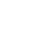# 什么是正则表达式？表达式定义字符串的搜索模式

1.1。什么是正则表达式？

2正则表达式的例子

.（点号）也是一个正则表达式，它匹配任何一个字符如：”a” 或 “1”。

this is text

this\s+is\s+text

^\d+(\.\d+)?

^ 定义了以什么开始

\d+ 匹配一个或多个数字

? 设置括号内的选项是可选的

\. 匹配 “.”

3.正则表达式规则

3.1。常见的匹配符号

[abc]

[abc][vz]

[^abc]

[a-d1-7]

X|Z

XZ

3.2。元字符

\d

\D

\s

\S

\w

\W

\S

\b

3.3。量化

X*X *0个或多个字母X，。*找到任何字符序列

X+ - 找到一个或几个字母X

X? 未找到或只有一个字母X

{X}

\d{3}搜索三位数字，.{10}对于长度为10的任何字符序列。

{X,Y}

X和Y之间发生，

\d{1,4}\d出现至少一次，并最多出现四个。

?后一个量词使其成为一个不贪婪的量词。它试图找到最小的匹配。。

3.4捕获组

``````String pattern = "(\\w)(\\s+)([\\.,])";
System.out.println(EXAMPLE_TEST.replaceAll(pattern, ""));``````

``````pattern = "(?i)()(.+?)()";
String updated = EXAMPLE_TEST.replaceAll(pattern, "\$2");``````

4Pattern 和Matcher

``````import java.util.regex.Matcher;
import java.util.regex.Pattern;
public class RegexTestPatternMatcher {
public static final String EXAMPLE_TEST = "This is my small example string which I'm going to use for pattern matching.";
public static void main(String[] args) {
Pattern pattern = Pattern.compile("\\w+");
// 如果您想忽略大小写敏感度,
// 你可以使用这个语句:
// Pattern pattern = Pattern.compile("\\s+", Pattern.CASE_INSENSITIVE);
Matcher matcher = pattern.matcher(EXAMPLE_TEST);
// 检查所有的结果
while (matcher.find()) {
System.out.print("Start index: " + matcher.start());
System.out.print(" End index: " + matcher.end() + " ");
System.out.println(matcher.group());
}
// 现在创建一个新的pattern和Matcher 以用选项卡替换空格s
Pattern replace = Pattern.compile("\\s+");
Matcher matcher2 = replace.matcher(EXAMPLE_TEST);
System.out.println(matcher2.replaceAll("\t"));
}
}``````

5Java正则表达式示例

``````public class LinkGetter {
private Pattern htmltag;
htmltag = Pattern.compile("]*href=\"[^>]*>(.*?)");
}
try {
String s;
StringBuilder builder = new StringBuilder();
builder.append(s);
}
Matcher tagmatch = htmltag.matcher(builder.toString());
while (tagmatch.find()) {
matcher.find();
.replaceFirst("\">", "")
.replaceFirst("\"[\\s]?target=\"[a-zA-Z_0-9]*", "");
}
}
} catch (MalformedURLException e) {
e.printStackTrace();
} catch (IOException e) {
e.printStackTrace();
}
}
private boolean valid(String s) {
if (s.matches("javascript:.*|mailto:.*")) {
return false;
}
return true;
}
private String makeAbsolute(String url, String link) {
}
return url + "/" + link;
}
return url + "/" + link;
}
}
}
throw new RuntimeException("Cannot make the link absolute. Url: " + url
}
}``````

``\b(\w+)\s+\1\b``

\ b是一个字边界，\ 1引用了第一个组的捕获匹配，即第一个字。 （？！ - in）\ b（\ w +）\ 1 \ b如果不以“-in”开头，则会找到重复的单词。 提示：添加（？s）以跨多行搜索

``(\n\s*)title``

``````
public class RegexTest {
public static void main( String args[] ){
// 按指定模式在字符串查找
String line = "My name is Jianguotang。I am a Android programmer.I am 21 years old";
String pattern = "(\\D*)(\\d+)(.*)";
// 创建 Pattern 对象
Pattern r = Pattern.compile(pattern);
// 现在创建 matcher 对象
Matcher m = r.matcher(line);
if (m.find( )) {
System.out.println("Found value: " + m.group(0) );
System.out.println("Found value: " + m.group(1) );
System.out.println("Found value: " + m.group(2) );
System.out.println("Found value: " + m.group(3) );
} else {
System.out.println("NO MATCH");
}
}
}
``````

``````Found value: My name is Jianguotang。I am a Android programmer.I am 21 years old
Found value: My name is Jianguotang。I am a Android programmer.I am
Found value: 21
Found value:  years old
``````

《什么是正则表达式？表达式定义字符串的搜索模式》